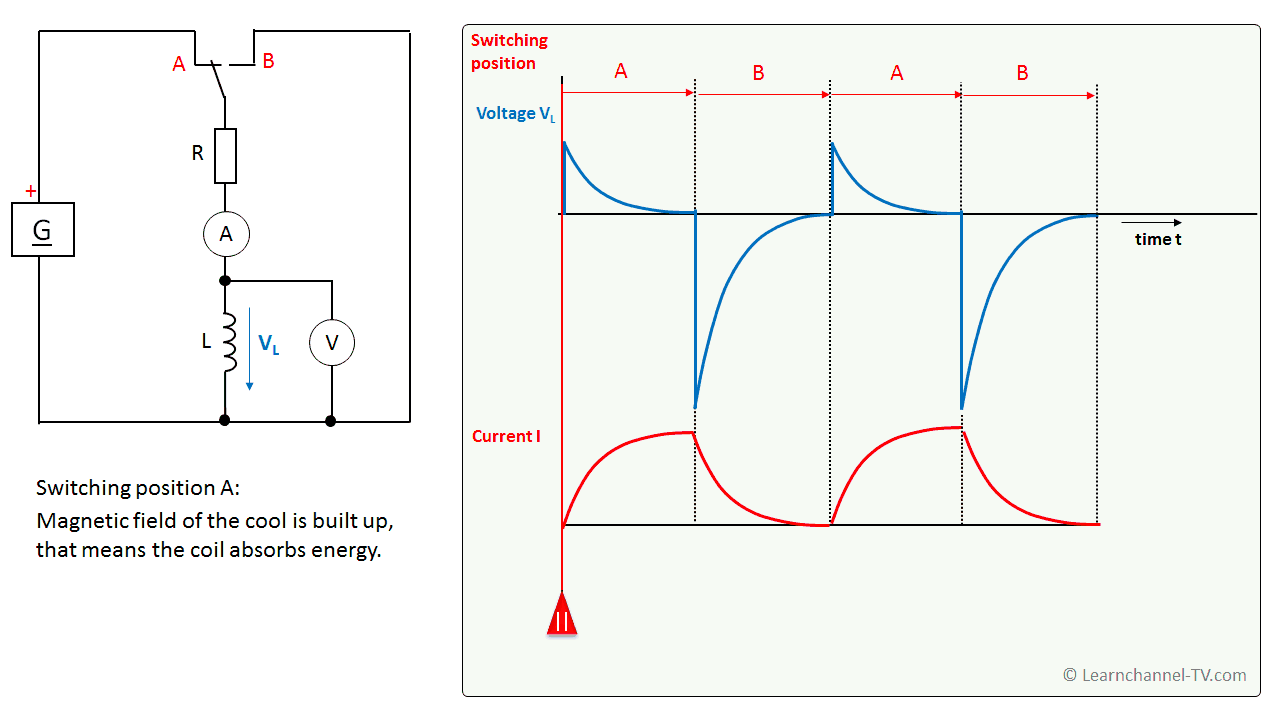# Indutores em circuitos de corrente contínua

(English)

## Inductors in DC Circuits

Test: The DC voltage at the coil is switched on and off. The voltage curve at the coil and the current are measured.Inductor in a DC Circuits

If the voltage at the coil is switched off, this means a large change in current. The coil reacts to this and induces a voltage, the so-called self-induction voltage, which counteracts the change in current. In other words: The coil tries to maintain the current flow.

A coil has an inductance L of 1 H (pronounced "Henry") when a self-induction voltage of 1 V is generated in one second with a constant change in current of 1 A.

Formula symbol Inductance: L Unit: Henry H or Ωs

Note: The term inductance is also generally used as an umbrella term for the coil.

Caution when switching off inductors! The self-induction voltage can be several times higher than the supply voltage!